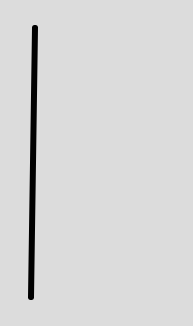p5.js | line() Function

The line() function is an inbuilt function in p5.js which is used to draw a line. In order to change the color of the line stroke() function is used and in order to change the width of the line strokeWeight() function is used.

Syntax:

line(x1, y1, x2, y2)

or

line(x1, y1, z1, x2, y2, z2)

Parameters: This function accepts six parameters as mentioned above and described below:

• x1: This parameter takes the x-coordinate of the first point.
• y1: This parameter takes the y-coordinate of the first point.
• z1: This parameter takes the z-coordinate of the first point.
• x2: This parameter takes the x-coordinate of the second point.
• y2: This parameter takes the y-coordinate of the second point.
• z2: This parameter takes the z-coordinate of the second point.

Below programs illustrates the line() function in P5.js:

Example 1: This example uses line() function to draw a line without using z-coordinate.

 function setup() {        // Set the canvas size      createCanvas(400, 400); }     function draw() {            // Set the background color     background(220);            // Set the stroke weight     strokeWeight(6);            //x1, y1 = 38, 31; x2, y2 = 300, 20;     // Use line() function to draw line     line(38, 31, 30, 200);  }

Output:Example 2: This example uses line() function to draw the line using z-coordinate.

 function setup() {        // Set the canvas size      createCanvas(400, 400); }     function draw() {            // Set the background color     background(220);            // Set the stroke weight     strokeWeight(6);            //x1, y1, z1 = 38, 31, 34;      // x2, y2, z2 = 300, 200, 45;     // Use line() function to draw line     line(38, 31, 34, 300, 200, 45);  }

Output:Reference: https://p5js.org/reference/#/p5/line

My Personal Notes arrow_drop_upCheck out this Author's contributed articles.

If you like GeeksforGeeks and would like to contribute, you can also write an article using contribute.geeksforgeeks.org or mail your article to contribute@geeksforgeeks.org. See your article appearing on the GeeksforGeeks main page and help other Geeks.

Please Improve this article if you find anything incorrect by clicking on the "Improve Article" button below.

Article Tags :

Be the First to upvote.

Please write to us at contribute@geeksforgeeks.org to report any issue with the above content.